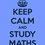# Help needed!!!!!

A person goes to sleep between 1am and 2 am and he wakes up when his watch shows such a time that the two hands interchange their respective places. He wakes up between 2am and 3am, how long does he sleep?

Consider only hour hand & minute hand.Note by Aneesh Kundu
6 years, 7 months ago

This discussion board is a place to discuss our Daily Challenges and the math and science related to those challenges. Explanations are more than just a solution — they should explain the steps and thinking strategies that you used to obtain the solution. Comments should further the discussion of math and science.

When posting on Brilliant:

• Use the emojis to react to an explanation, whether you're congratulating a job well done , or just really confused .
• Ask specific questions about the challenge or the steps in somebody's explanation. Well-posed questions can add a lot to the discussion, but posting "I don't understand!" doesn't help anyone.
• Try to contribute something new to the discussion, whether it is an extension, generalization or other idea related to the challenge.
• Stay on topic — we're all here to learn more about math and science, not to hear about your favorite get-rich-quick scheme or current world events.

MarkdownAppears as
*italics* or _italics_ italics
**bold** or __bold__ bold
- bulleted- list
• bulleted
• list
1. numbered2. list
1. numbered
2. list
Note: you must add a full line of space before and after lists for them to show up correctly
paragraph 1paragraph 2

paragraph 1

paragraph 2

[example link](https://brilliant.org)example link
> This is a quote
This is a quote
    # I indented these lines
# 4 spaces, and now they show
# up as a code block.

print "hello world"
# I indented these lines
# 4 spaces, and now they show
# up as a code block.

print "hello world"
MathAppears as
Remember to wrap math in $$ ... $$ or $ ... $ to ensure proper formatting.
2 \times 3 $2 \times 3$
2^{34} $2^{34}$
a_{i-1} $a_{i-1}$
\frac{2}{3} $\frac{2}{3}$
\sqrt{2} $\sqrt{2}$
\sum_{i=1}^3 $\sum_{i=1}^3$
\sin \theta $\sin \theta$
\boxed{123} $\boxed{123}$

## Comments

Sort by:

Top Newest

The answer is $\boxed {\frac{12}{13}}$ hours.

The positions of hour-hand and minute-hand have a certain relationship. They are together at 00:00h or 12:00AM. Let 12:00AM position be $0^\circ$, the time the person goes to sleep at $t_1$ and wakes up at $t_2$, the angle made by the hour-hand at $t_1$ and minute-hand at $t_2$ be $\alpha$ and that by the hour-hand at $t_2$ and minute-hand at $t_1$ be $\beta$. Note that the hour-hand makes $30^\circ$ on the dial in $1$ hour while the minute-hand makes $360^\circ$ in $1$ hour.

Therefore, we have $\quad 30t_1 = \alpha \space, \quad 360t_1 = 360 + \beta$ (as $t_1 > 1$ hour) $\quad \Rightarrow t_1 = \dfrac {\alpha}{30} = 1 + \dfrac {\beta}{360}$

Similarly, we have $\quad t_2 = \dfrac {\beta}{30} = 2 + \dfrac {\alpha}{360}$

The time that the person sleep, $t = t_2-t_1 = \dfrac {\beta-\alpha} {30} = 1 - \dfrac {\beta - \alpha}{360}$

$\dfrac {\beta-\alpha} {30} \left( 1 + \dfrac {1}{12} \right) = 1\quad \Rightarrow \dfrac {\beta-\alpha} {30} = t = \boxed{\frac {12}{13}}$ hours.

- 6 years, 7 months ago

Log in to reply

thanx a lot!!

- 6 years, 7 months ago

Log in to reply

Why you add 10 to y in the equation 10+y/60

- 4 years, 7 months ago

Log in to reply

he slept at 1:10 and woke up at 2:05

- 6 years, 7 months ago

Log in to reply

According to the book the answer should be $55\dfrac{5}{13}\text{ mins}$

- 6 years, 7 months ago

Log in to reply

but the question says that consider minute and hour hand only

- 6 years, 7 months ago

Log in to reply

yes but that doesn't mean that time can't be fractional

- 6 years, 7 months ago

Log in to reply

A similar question once appeared in INMO And I really think this is a gud one So pls reshare and write solutions

- 6 years, 7 months ago

Log in to reply

The book is correct. Let $x$ and $y$ be the minutes past $1 am$ and $2 am$ where the hands could be. Then we solve this system of equations, for start and end times, the difference being the time of sleeping:

$\dfrac { 10+y }{ 60 } =\dfrac { x }{ 5 }$

$\dfrac { 5+x }{ 60 } =\dfrac { y }{ 5 }$

We get $x=\dfrac { 125 }{ 143 }$ and $y=\dfrac { 70 }{ 143 }$, from which we can work out the time of sleep

$(120+5+x)-(60+10+y)=\dfrac { 720 }{ 13 } =55+\dfrac { 5 }{ 13 }$

- 6 years, 7 months ago

Log in to reply

Thanx a lot!!

- 6 years, 7 months ago

Log in to reply

Why u add 10 to y in the equation (10+y)/60

- 4 years, 7 months ago

Log in to reply

×

Problem Loading...

Note Loading...

Set Loading...